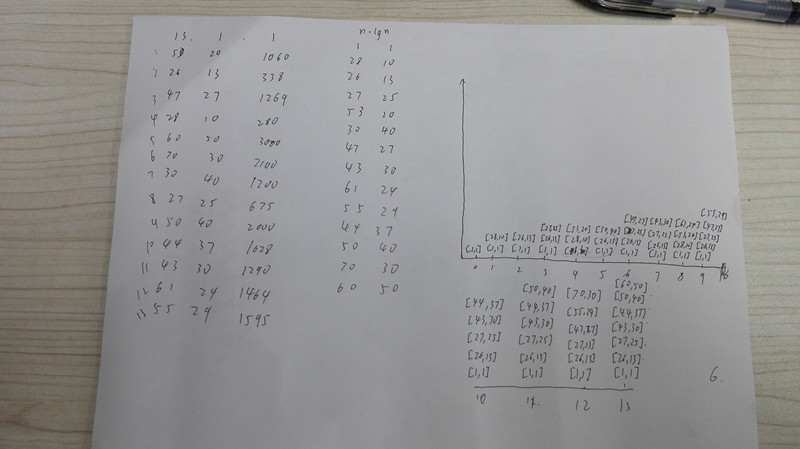编码-京东实习笔试编程题-生日礼物-动态规划import java.util.ArrayList;
import java.util.Arrays;
import java.util.Comparator;
import java.util.List;
import java.util.Scanner;

public static void main(String[] args) {
Scanner scanner = new Scanner(System.in);
while(scanner.hasNextInt()){
int num = scanner.nextInt();
int w = scanner.nextInt();
int h = scanner.nextInt();
int[][] temps = new int[num];
int nums = 0;
for (int i = 0; i < num; i++) {
int tempWidth=scanner.nextInt();
int tempHigh=scanner.nextInt();
if (tempWidth>w && tempHigh>h) {
temps[nums] = tempWidth;
temps[nums] = tempHigh;
temps[nums] = i+1;
temps[nums] = temps[nums]*temps[nums];
nums++;
}
}
findTemp(Arrays.copyOfRange(temps, 0, nums),nums,w,h);
}
}

public static void findTemp(int[][] temps, int num, int w, int h) {
//首先对所有的数据进行排序，以面积的形式进行排序
Arrays.sort(temps,new Comparator<int[]>() {
@Override
public int compare(int[] o1, int[] o2) {
if (o1>o2) return 1;
if (o1<o2) return -1;
return 0;
}
});
List<List<int[]>> resultList = new ArrayList<>();
for (int i = 1; i <= num; i++) {
int levelNow = 0;
for (int j = i-1; j >= 0; j--) {
List<int[]> list = resultList.get(j);
int oldLevel = list.size();
if(oldLevel<levelNow-1) break;
int[] oldTop = list.get(oldLevel-1);
if (oldTop<temps[i-1] && oldTop<temps[i-1]) {
List<int[]> tempList = new ArrayList<>();
//更新resultList，保存最优值
if (resultList.size()==i+1) resultList.remove(i);
levelNow = oldLevel+1;
}
}
}
System.out.println("最多可以包裹的信封数:\t"+(resultList.get(num).size()-1));
for (int i = 1; i < resultList.get(num).size(); i++) {
System.out.print(resultList.get(num).get(i)+" ");
}
}
}